Root

Root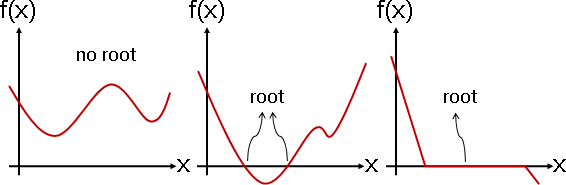```          _________
-b ± V b² - 4ac
x = ―――――――――――――――
2a
```

Root: Bisection Method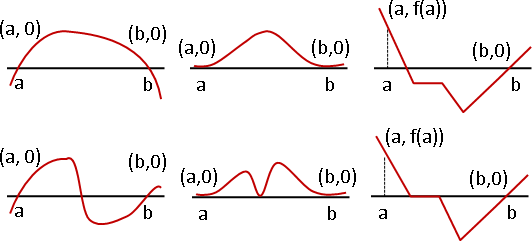f(a)和f(b)同號的區間，放棄處理，不確定是否有根。f(a)和f(b)為零的區間，中止處理，顯然已經找到一個根。f(a)和f(b)異號的區間，繼續處理，二分尋找根。

float變數、double變數，能夠儲存的位數有限，不可能精確，有著一個極限。不斷分割區間，就能到達極限。一個float變數的範圍約為10³⁸到10⁻³⁸，分割區間log₂10⁷⁶ ≈ 252次，定能得到float變數所能儲存的最精確的數值。

[a,b]寬度大於自訂精確度，就繼續分割區間。[a,b]寬度小於等於自訂精確度，就立即停止迴圈。因為c永遠在[a,b]當中，所以c的誤差不超過自訂精確度。Root: Newton's Method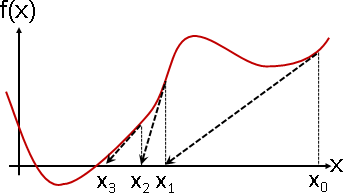```x = sqrt(n)
x² = n
x² - n = 0
f(x) = x² - n
f′(x) = 2x
```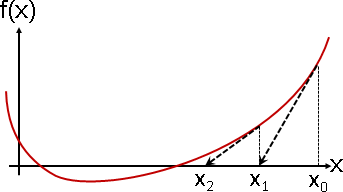Root: Secant MethodFixed Point

Fixed Point（Fixpoint）Eigenpoint【尚無正式名稱】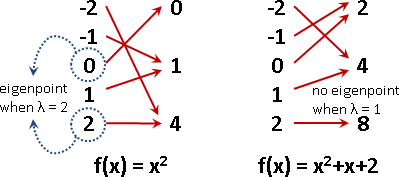```f(x) = 0   <--->   f(x) + x = x   <--->   g(x) = x
```

```f(x) = 0   <--->   λ (f(x) + x) = λx   <--->   h(x) = λx
```

Fixed Point: Fixed Point Iteration```|x₁ - x₀| > |x₂ - x₁| > |x₃ - x₂| > ......

|x₁ - x₀| > |x₂ - x₁|   此處以開頭兩項為例。事實上任意相鄰兩項都成立。

|x₁ - x₀| > |f(x₁) - f(x₀)|

|f(x₁) - f(x₀)|
1 > ——————————————— = |slope|
|x₁ - x₀|

|slope| < 1

-1 < slope < 1
```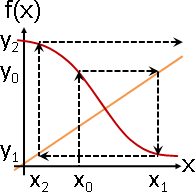```|x₁ - x₀| > |x₂ - x₁| > |x₃ - x₂| > ......

|x₁ - x₀| > |x₂ - x₁|   此處以開頭兩項為例。事實上任意相鄰兩項都成立。

|x₁ - x₀| > |f(x₁/λ) - f(x₀/λ)|   上次求得的數值，除以λ之後才代入。

|λχ₁ - λχ₀| > |f(χ₁) - f(χ₀)|     變數代換

|λ| |χ₁ - χ₀| > |f(χ₁) - f(χ₀)|

|f(χ₁) - f(χ₀)|
|λ| > ——————————————— = |slope|
|χ₁ - χ₀|

|slope| < |λ|

-|λ| < slope < |λ|               介於±λ
```

Equation

Equation

```2x + 1 = (4x² + 3) / (x - 1)
``````2x + 1 = (4x² + 3) / (x - 1)

2x + 1 - (4x² + 3) / (x - 1) = 0

f(x) = 2x + 1 - (4x² + 3) / (x - 1)
```

```f(x) = 0                                  root
f(x) + x = x          --->  g(x) = x      fixed point
λf(x) + λx = λx       --->  h(x) = λx     eigenpoint
λf(x) + λq(x) = q(x)  --->  p(x) = q(x)   solution
```

UVa 358 10341 10428 10566 10668 11277Increasing Function：遞增函數。輸入變大，輸出跟著變大（或不變）。斜率是正數（或零）。適合Bisection Method。

Convex Function：凸函數。隨便劃一道割線，函數曲線總是凹下去（或平貼）。斜率是遞增函數。適合Newton's Method。

Lipschitz Function：平緩函數。隨便劃一道割線，斜率的絕對值小於（或等於）一個定值。適合Fixed Point Iteration。

Polynomial Function：多項式函數。可以手工推導公式解，也可以使用上述演算法求解。

System of Equations（Simultaneous Equations）

```{ 1 x + 2 y - 4 sin(z) = 1
{ 2 x - 4 y + 2 cos(z) = 2
{ 3 x + 1 y + 1 exp(z) = 3
```

Inequality

Inequality（Inequation）

「不等式」。等號=改成了小於<、大於>、小於等於≤、大於等於≥、不等於≠。

```2x + 1 < (4x² + 3) / (x - 1)
```

System of Inequalities

「不等式組」、「聯立不等式」。許多道不等式同時成立。

```{ 1 x + 2 y - 4 sin(z) < 1
{ 2 x - 4 y + 2 cos(z) ≤ 2
{ 3 x + 1 y + 1 exp(z) > 3
```

```{  1 x + 2 y - 4 sin(z) - 1 < 0          { f(x,y,z) < 0
{  2 x - 4 y + 2 cos(z) - 2 ≤ 0   --->   { g(x,y,z) ≤ 0
{ -3 x - 1 y - 1 exp(z) + 3 < 0          { h(x,y,z) < 0
```

Constraint Satisfaction

「約束滿足」。許多道等式與不等式同時成立。